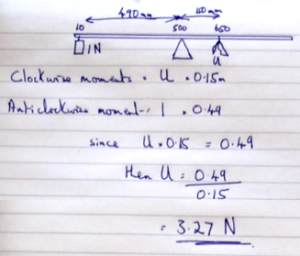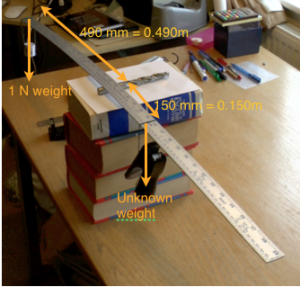### Law of moments calculation

The principle allows you to calculate the value of an unknown force or mass. This idea is used in, for example, a weigh bridge for vehicles or the operation of a crane. On this page however we stick to basic examples with calculations.Calculation using the Law of Moments